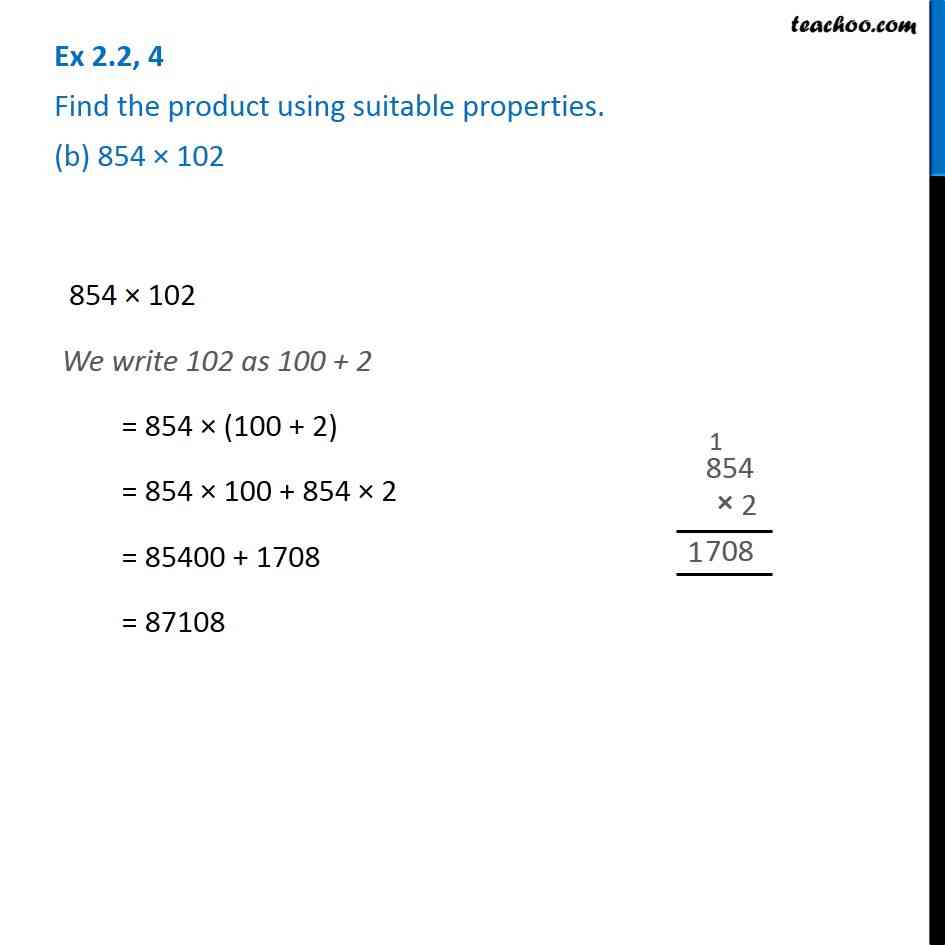1. Chapter 2 Class 6 Whole Numbers
2. Serial order wise
3. Ex 2.2

Transcript

Ex 2.2, 4 Find the product using suitable properties. (b) 854 × 102 854 × 102 We write 102 as 100 + 2 = 854 × (100 + 2) = 854 × 100 + 854 × 2 = 85400 + 1708 = 87108